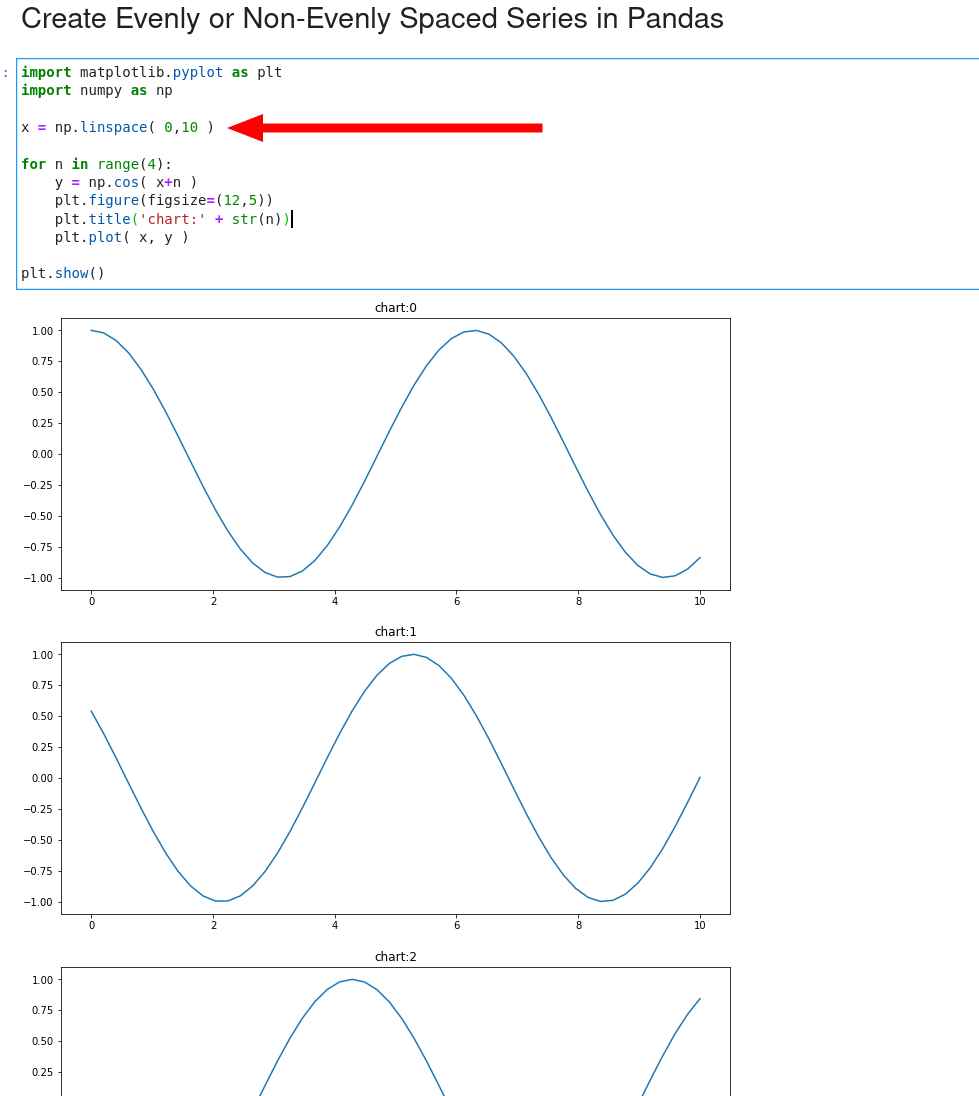In this short tutorial, we'll see how to create evenly or unevenly spaced arrays/series in Pandas and Python. To create evenly spaced arrays we will use numpy methods: `np.linspace()` and `np.logspace()`.

(1) evenly spaced arrays/series

``````np.linspace(1, 5)
``````

(2) non-evenly spaced arrays/series

``````np.logspace(0, 1, 3)
np.geomspace(1,3,5)
``````

## 1. Create evenly spaced arrays in Python

To create evenly spaced arrays in Python we can use the method: `np.linspace()`. It has 3 important parameters:

• `start` - The starting value of the sequence.
• `stop` - The end value of the sequence, unless endpoint is set to False.
• `num` - Number of samples to generate. Default is 50. Must be non-negative.

Some examples of evenly spaced arrays:

``````import numpy as np
np.linspace(0, 5, 10)
``````

result:

``````array([0.        , 0.55555556, 1.11111111, 1.66666667, 2.22222222,
2.77777778, 3.33333333, 3.88888889, 4.44444444, 5.        ])
``````
``````np.linspace(1, 5)
``````

result:

``````array([1.        , 1.08163265, 1.16326531, 1.24489796, 1.32653061,
1.40816327, 1.48979592, 1.57142857, 1.65306122, 1.73469388,
1.81632653, 1.89795918, 1.97959184, 2.06122449, 2.14285714,
2.2244898 , 2.30612245, 2.3877551 , 2.46938776, 2.55102041,
2.63265306, 2.71428571, 2.79591837, 2.87755102, 2.95918367,
3.04081633, 3.12244898, 3.20408163, 3.28571429, 3.36734694,
3.44897959, 3.53061224, 3.6122449 , 3.69387755, 3.7755102 ,
3.85714286, 3.93877551, 4.02040816, 4.10204082, 4.18367347,
4.26530612, 4.34693878, 4.42857143, 4.51020408, 4.59183673,
4.67346939, 4.75510204, 4.83673469, 4.91836735, 5.        ])
``````

The official documentation is placed on: numpy.linspace

## 2. Create math functions with np.linspace()

We can use `np.linspace()` to generate mathematical functions like:

• sin
• cos etc

Below we can find visualization of math cos in Python and Matplotlib:

``````import matplotlib.pyplot as plt
import numpy as np

x = np.linspace( 0,10 )

for n in range(4):
y = np.cos( x+n )
plt.figure(figsize=(12,5))
plt.title('chart:' + str(n))
plt.plot( x, y )

plt.show()
``````

the result is plot of `cos` function:## 3. Create unevenly spaced arrays in Python

To make unevenly spaced arrays in Python and Pandas we can use several numpy functions like:

• `np.geomspace()`
• `np.logspace()`

Two examples of them:

``````np.geomspace(1,16,5)
``````

will produce non evenly spaced array with 5 items from 1 to 16:

``````array([ 1.,  2.,  4.,  8., 16.])
``````

While:

``````np.logspace(0, 2, 3)
``````

will produce non evenly spaced array with 3 items from 1 to 100:

``````array([  1.,  10., 100.])
``````

In some cases the output is in scientific notation:

``````np.logspace(0, 4, 3)
``````

result:

``````array([1.e+00, 1.e+02, 1.e+04])
``````

## 4. Make DataFrames with Uneven Array Lengths

Finally let's cover a topic -** how to create a DataFrame from non-even array length.**

If we try to create DataFrame from arrays with different length we will get an error:

``````import pandas as pd
data = {"a": [1, 2, 3],
"b": [1, 2, 3, 4]}
pd.DataFrame(data)
``````

the result is:

``````ValueError: All arrays must be of the same length
``````

We can solve the error by method `from_dict` with parameter `orient='index'`:

``````pd.DataFrame.from_dict(data, orient='index').T
``````

the result would be:

a b
0 1.0 1.0
1 2.0 2.0
2 3.0 3.0
3 NaN 4.0

## Conclusion

We saw how to create evenly spaced arrays, simulate mathematical functions and visualize them. It was discussed how to generate non evenly spaced arrays by multiple functions.

Finally we covered how to solve error - ValueError: All arrays must be of the same length - and create DataFrame from non-evenly arrays lengths.